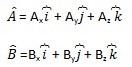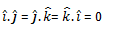Checkout JEE MAINS 2022 Question Paper Analysis : Checkout JEE MAINS 2022 Question Paper Analysis :

# The scalar Product: Product Of Two Vectors

Many physical quantities we deal with are represented as vector quantities, such as velocity, force, etc. These quantities interact with each other to produce a resultant effect. In order to find the resultant of these forces, operations such as addition, subtraction, and multiplication are required to be performed by these forces. In this section, we will learn about the multiplication of two vector quantities.

There are two types of vector products possible; the scalar multiplication, which produces a scalar as the product of the multiplication, and the other is vector multiplication, which produces a vector as a product. Here we will learn about the scalar product of two vectors.

Scalar Product

Let us consider two vectors A and B. The dot product of these two vectors is given as, Where is the angle between these two vectors?

The scalar product can also be written as,As we know BcosƟ is the projection of B onto A and AcosƟ is the projection of A on B, the scalar product can be defined as the product of the magnitude of A and the component of B along with A or the product of the magnitude of A and the component of B along with A.

Commutative lawDistributive LawWhere λ is a real number.

Let us discuss the dot product of two vectors in three-dimensional motion. Consider two vectors represented in terms of three unit vectors,Where, is the unit vector along the x-direction,  is the unit vector along the y-direction and is the unit vector along the z-direction.

The scalar product of the two vectors is given by,Here,## Direction Cosines and Direction Ratios of a Line## Frequently Asked Questions – FAQs

### What is the meaning of a vector quantity?

A vector quantity is a quantity that has both magnitudes as well as a distinct direction.

### What is the meaning of a scalar quantity?

A scalar quantity is a quantity that has only magnitude.

### Give a few examples of scalar quantities.

Density, volume, speed, time, energy, and mass are some of the most familiar examples of scalar quantities.

### Give a few examples of vector quantities.

Velocity, displacement, force, weight, and acceleration are some of the most common examples of vector quantities.

### What are the two types of vector products?

There are two types of vector products possible; scalar multiplication, which produces a scalar as the product of the multiplication, and the other is vector multiplication, which produces a vector as a product.
Test your Knowledge on Scalar Product